# 4 Digit Subtraction with Regrouping

4 Digit Subtraction with Regrouping – Question 1

Subtract the following: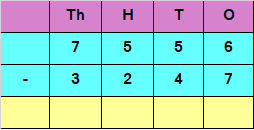Explanation

If we want to Subtract the digits at ones place, we have

6 – 7

But 7 is greater than 6

We would need to borrow 1 from 5 in tens place

6 in ones place would now become 16 and 5 in tens place would be reduced to 4

So, 16 – 7 = 9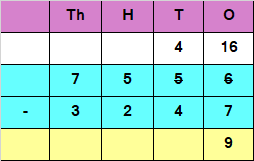Subtracting the digits at tens place

4 – 4 = 0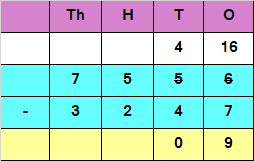Subtracting the digits at hundreds place

5 – 2 = 3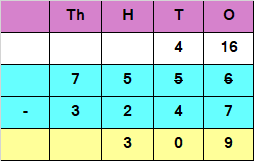Subtracting the digits at thousands place

7 – 3 = 4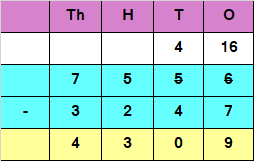Hence, the difference is 4309

4 Digit Subtraction with Regrouping – Question 2

Subtract the following: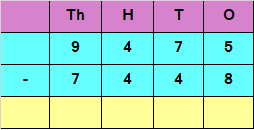Explanation

If we want to Subtract the digits at ones place, we have

5 – 8

But 8 is greater than 5

We would need to borrow 1 from 7 in tens place

5 in ones place would now become 15 and 7 in tens place would be reduced to 6

So, 15 – 8 = 7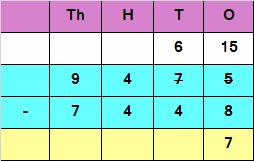Subtracting the digits at tens place

6 – 4 = 2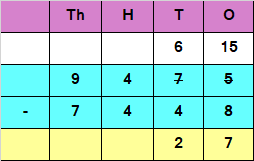Subtracting the digits at hundreds place

4 – 4 = 0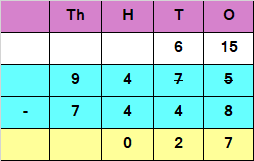Subtracting the digits at thousands place

9 – 7 = 2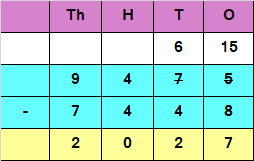Hence, the difference is 2027